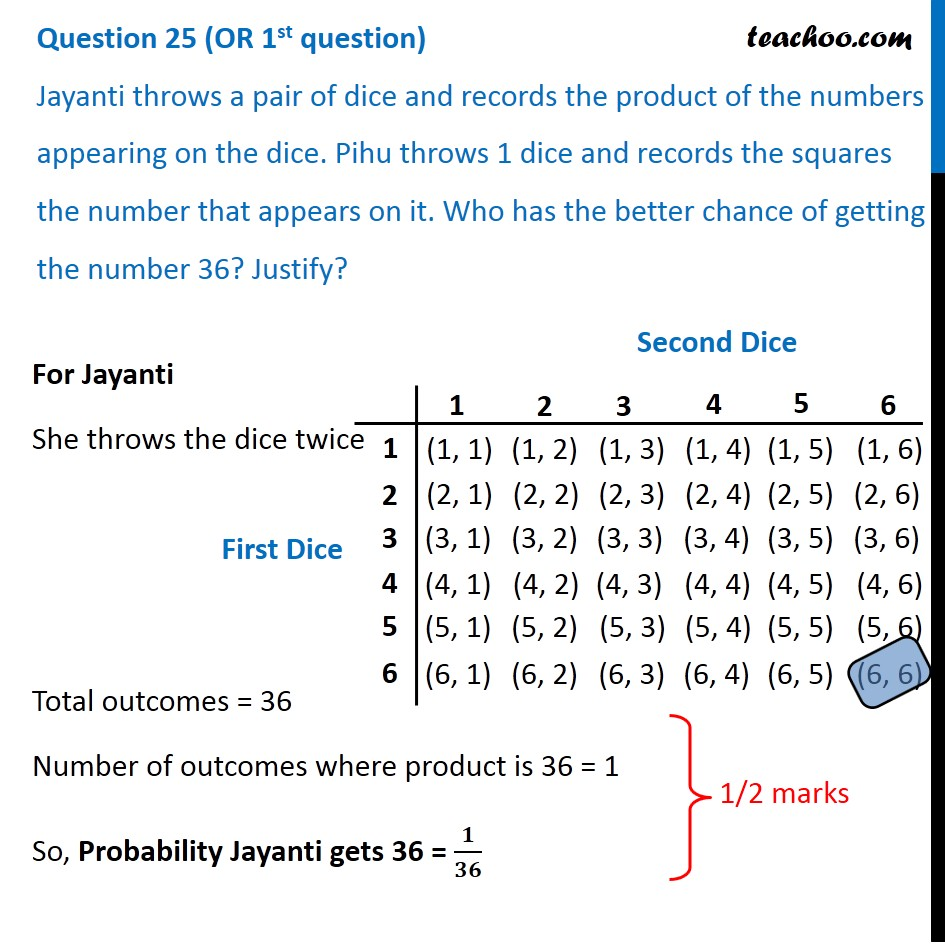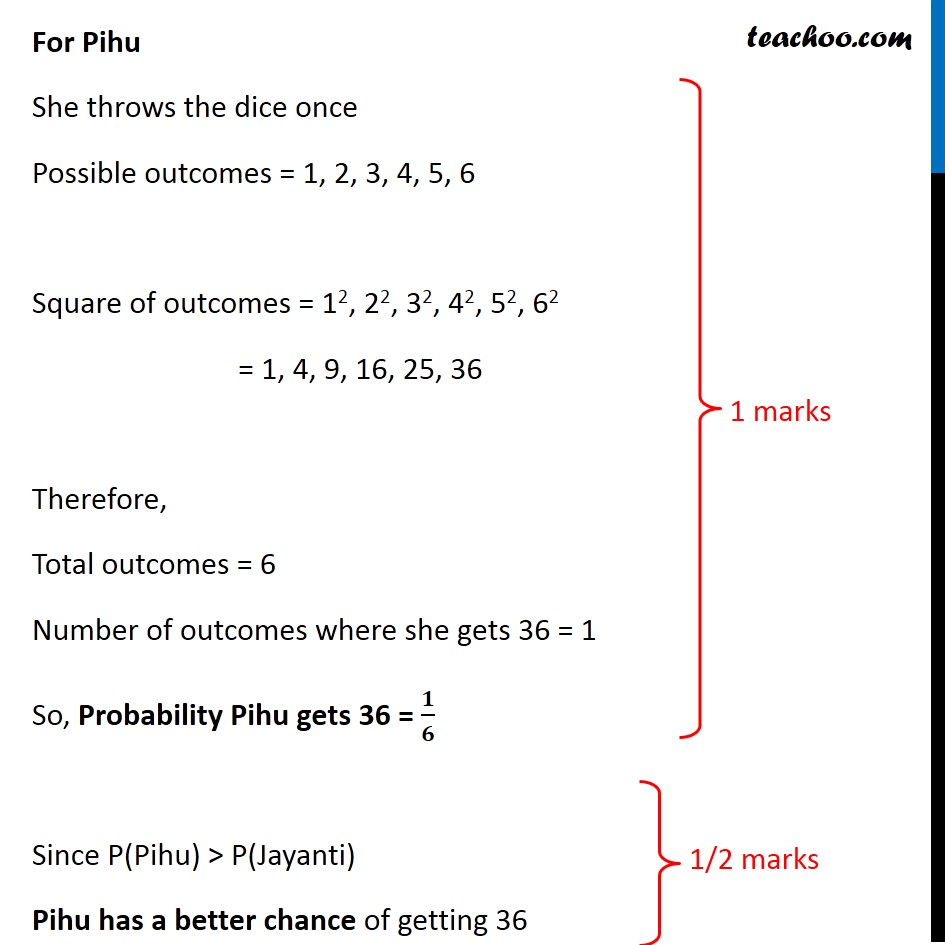CBSE Class 10 Sample Paper for 2020 Boards - Maths Standard

Class 10
Solutions of Sample Papers for Class 10 Boards

## Jayanti throws a pair of dice and records the product of the numbers appearing on the dice. Pihu throws 1 dice and records the squares the number that appears on it. Who has the better chance of getting the number 36? Justify?### Transcript

Question 25 (OR 1st question) Jayanti throws a pair of dice and records the product of the numbers appearing on the dice. Pihu throws 1 dice and records the squares the number that appears on it. Who has the better chance of getting the number 36? Justify? For Jayanti She throws the dice twice Total outcomes = 36 Number of outcomes where product is 36 = 1 So, Probability Jayanti gets 36 = 𝟏/𝟑𝟔 For Pihu She throws the dice once Possible outcomes = 1, 2, 3, 4, 5, 6 Square of outcomes = 12, 22, 32, 42, 52, 62 = 1, 4, 9, 16, 25, 36 Therefore, Total outcomes = 6 Number of outcomes where she gets 36 = 1 So, Probability Pihu gets 36 = 𝟏/𝟔 Since P(Pihu) > P(Jayanti) Pihu has a better chance of getting 36